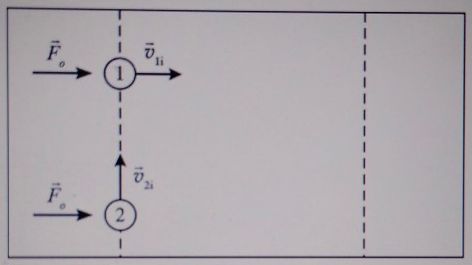# Problem: Two identical pucks slide across a level, frictionless table. Initially, the pucks have the same speed, but their velocities are perpendicular to each other as shown. The same constant force is exerted on each puck for the same interval of time: from the instant shown until puck 1 crosses the second dotted line. The pucks remain on the table and do not collide during this time interval.(a) The magnitude of the change in momentum of puck 1 is _ the magnitude of the change in momentum of puck 2.(b) The magnitude of the final momentum of puck 1 is _ the magnitude of the final momentum of puck 2.

###### FREE Expert Solution

This problem involves changes in momentum and a constant applied force.

Momentum-impulse relation:

$\overline{){\mathbf{∆}}{\mathbf{p}}{\mathbf{=}}{\mathbf{m}}{\mathbf{∆}}{\mathbf{v}}{\mathbf{=}}{\mathbf{F}}{\mathbf{∆}}{\mathbf{t}}}$

90% (137 ratings)###### Problem Details

Two identical pucks slide across a level, frictionless table. Initially, the pucks have the same speed, but their velocities are perpendicular to each other as shown. The same constant force is exerted on each puck for the same interval of time: from the instant shown until puck 1 crosses the second dotted line. The pucks remain on the table and do not collide during this time interval.(a) The magnitude of the change in momentum of puck 1 is _ the magnitude of the change in momentum of puck 2.
(b) The magnitude of the final momentum of puck 1 is _ the magnitude of the final momentum of puck 2.